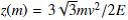### Ordinary Versus Novel Particle With the Example of Their Spontaneous Transition

•  Josip Soln

#### Abstract

The bicubic equation of particle limiting velocity formalism yields three solutions c1, c2 and c3, (primary, secondary and tertiary) limiting velocities in terms of the congruent parameterwhich is defined in terms of m, v, and E, respectively being particle mass, velocity and energy. The bicubic equation discriminant D is given in terms of the congruent parameter z(m). When one has z2(m) ≤ 1 with the discriminant satisfying D ≤ 0 then we are talking about limiting velocities of ordinary particles. Good examples are the relativistic particles such as electron, neutrino,etc., with luminal limiting velocity c3 = c and calculated superluminal c2, and imaginary superluminal c1, all corresponding to the real particle energy. On the specific level, the situations like these, we discuss in the muon neutrino velocities with the OPERA detector and the electron velocities from the 2010 Grab Nebula Flare. The z(m) = 1 value separates the ordinary particles from novel particles, associated with D ⪰ 0 and z2 ⪰ 1 with new novel particle limiting velocity solutions c1, c2 and c3 which depend, in addition to z(m), also on the congruent angle α(m), nonlinearly related to z(m). These solutions are discussed on the newly defined sterile neutrino which here is modeled as an ordinary particle with z2 ⪯ 1 spontaneously transiting via z(m) = 1 into the modeled novel sterile neutrino with z2 ⪰ 1. All ordinary and novel particles limiting velocities carry real particle energies; the ordinary particle limiting velocity solutions being in quadratic forms, while the novel particle limiting velocity solutions being respectively, in quadratic complex form, linear complex form, and just congruent angle α complex quadratic form.This work is licensed under a Creative Commons Attribution 4.0 License.
• ISSN(Print): 1916-9639
• ISSN(Online): 1916-9647
• Started: 2009
• Frequency: semiannual